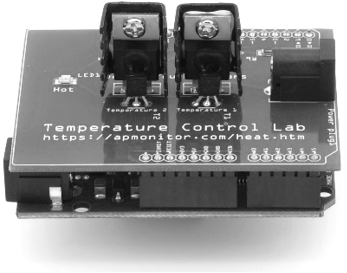## Quiz: Solve ODEs with Python1. Which input is not necessary for the ODEINT function from Scipy.Integrate?

A. A function that returns derivatives when given states (y) and time (t)
Incorrect. model is required for y = odeint(model, y0, t)
B. Boundary conditions
Correct. Boundary (spatial) conditions are used in the solution of partial differential equations and are not solved with an ordinary differential equation solver such as ODEINT.
C. Initial Conditions
Incorrect. y0 is required for y = odeint(model, y0, t)
D. Time points
Incorrect. t is required for y = odeint(model, y0, t)

2. What will an increased value of k do to the value of y(3) (the value of y at time=3) with initial condition y(0)=5?

$$\frac{dy}{dt}=-k \, y$$

import numpy as np
from scipy.integrate import odeint
def model(y,t):
k = 0.3
return -k * y
y = odeint(model,5,[0,3])
A. Increase y(3)
Incorrect. Try the code and observe the value of y at time=3. A higher value of k causes a faster decay to zero.
B. Decrease y(3)
Correct. A higher value of k causes a faster decay to zero.
C. The value of k has no effect on y(3)
Incorrect. Try the code and observe the value of y at time=3. A higher value of k causes a faster decay to zero.

3. The differential equation dy/dt=-0.3y is integrated in 0.1 time steps to a final time of 3.0 with initial condition y(0)=5.

import numpy as np
from scipy.integrate import odeint
n=31 # total time points
def model(y,t):
return -0.3 * y
y=np.empty(n); t=np.empty(n)
for i in range(n):
if i==0:
t[i] = 0.0  # initial time
y[i] = 5    # initial condition
else:
# integrate forward with time-step 0.1
t[i] = t[i-1]+0.1
y[i] = odeint(model,y[i-1],[t[i-1],t[i]])[-1]

What is the correct way to print the value of y at time=1.5?

A. print(y[1.5])
Incorrect. The command print(y[1.5]) gives a syntax error because y must be indexed with an integer, not a floating point number. Each time step is 0.1.
B. print(y[-1])
Incorrect. The value y[-1] is the last in the list, corresponding to time=3.0.
C. print(y)
Incorrect. The value y corresponds to time=1.6.
D. print(y)
Correct. The value y corresponds to time=1.5.